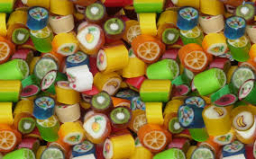# Conditional probability

Suppose a batch contains ten items, of which four are defective. Two items are drawn at random from the batch, one after the other, without replacement. What is the probability that:
I) both are defective?
Ii) Is the second item defective?

p1 =  0.1333
p2 =  0.4

### Step-by-step explanation:Did you find an error or inaccuracy? Feel free to write us. Thank you!

Tips for related online calculators
Need help calculating sum, simplifying, or multiplying fractions? Try our fraction calculator.
Would you like to compute the count of combinations?